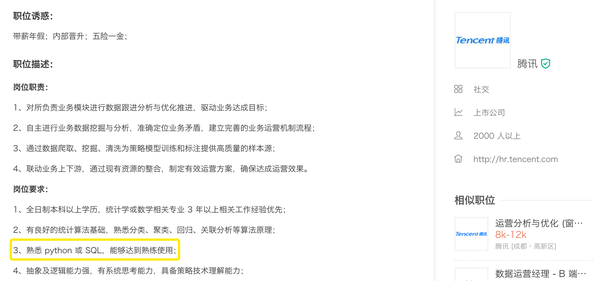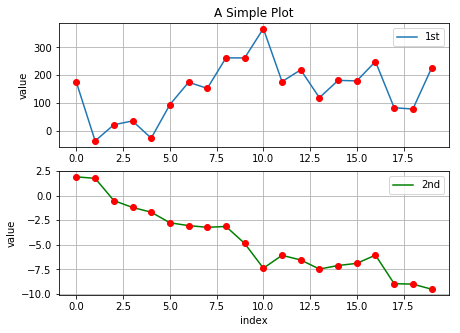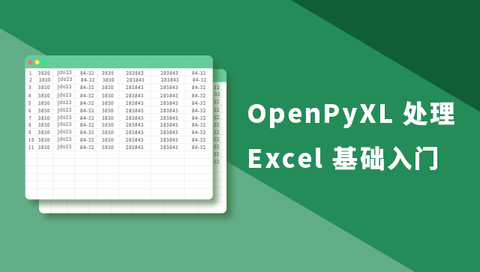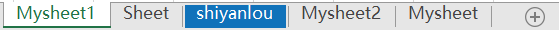# 用 Python 读写 Excel 表格，就是这么的简单粗暴且乏味 - 知乎

2020/04/10 12:15Python 究竟有什么法力能让大家如此青睐？OpenPyXL 处理 Excel 基础入门www.shiyanlou.com• 创建/打开工作簿
• 访问工作表单元及其值
• 保存工作表

### 创建/打开工作簿

!wget -nc "https://labfile.oss.aliyuncs.com/courses/1585/shiyanlou.xlsx"
!pip install openpyxl==3.0.3

from openpyxl import Workbook

wb = Workbook()  # 实例化一个工作簿对象

print(wb)

from openpyxl import load_workbook

print(wb)

load_workbook 中可以使用以下几个参数：

• data_only：带有公式的单元格是否具有公式（默认具有）或上一次 Excel 读取工作表时存储的值。
• keep_vba：设置是否保留任何 Visual Basic 元素（默认保留），可选择保留但是不支持编辑。

ws = wb.active  # 获取当前活跃的工作表
print(ws)

ws = wb.create_sheet() # sheet
ws1 = wb.create_sheet("Mysheet")  # 命名为 Mysheet
ws2 = wb.create_sheet("Mysheet1", 0)  # 新建 Mysheet1 工作表插入到第一个位置
ws3 = wb.create_sheet("Mysheet2", -1)  # 新建 Mysheet2 工作表插入到倒数第二个的位置
ws.title = "shiyanlou"  # 将上述 ws 工作表重命名为 shiyanlou

print("Success")

ws.sheet_properties.tabColor = "1072BA"  # RGB 格式
print(ws.sheet_properties.tabColor)ws = wb["shiyanlou"]
print(ws)

print(wb.sheetnames)

for sheet in wb:
print(sheet.title)

source = wb.active  # 获取活跃的工作表
target = wb.copy_worksheet(source)  # 上述获取的工作表为其创建副本

print(target)

### 访问工作表单元及其值

c = ws['A4']  # 将访问 A4 单元格
ws['A4'] = 4  # 对 A4 单元格进行赋值

c.value  # 访问 A4 单元格的值，同 ws['A4'].value

d = ws.cell(row=4, column=2, value=10)  #  B4 进行赋值

ws['B4'].value  # 访问单元格的值

### 访问多个单元格

cell_range = ws['A1':'C2']

cell_range

# 访问列
colC = ws['C']
col_range = ws['C:D']

# 访问行
row10 = ws
row_range = ws[5:10]

print(col_range, row_range)

for row in ws.iter_rows(min_row=1, max_col=3, max_row=2):
for cell_row in row:
print(cell_row)

for col in ws.iter_cols(min_row=1, max_col=3, max_row=2):
for cell_col in col:
print(cell_col)

ws['C9'] = 'hello world'
tuple(ws.rows)

tuple(ws.columns)

### 访问值

for row in ws.values:
for value in row:
print(value)

Worksheet.iter_rows()Worksheet.iter_cols() 可以用 values_only 参数，只返回单元格的值：

for row in ws.iter_rows(min_row=1, max_col=3, max_row=2, values_only=True):
print(row)

### 保存工作表

wb.save("shiyanlou.xlsx")

OpenPyXL 处理 Excel 基础入门www.shiyanlou.com0
0 收藏

### 作者的其它热门文章0 评论
0 收藏
0